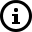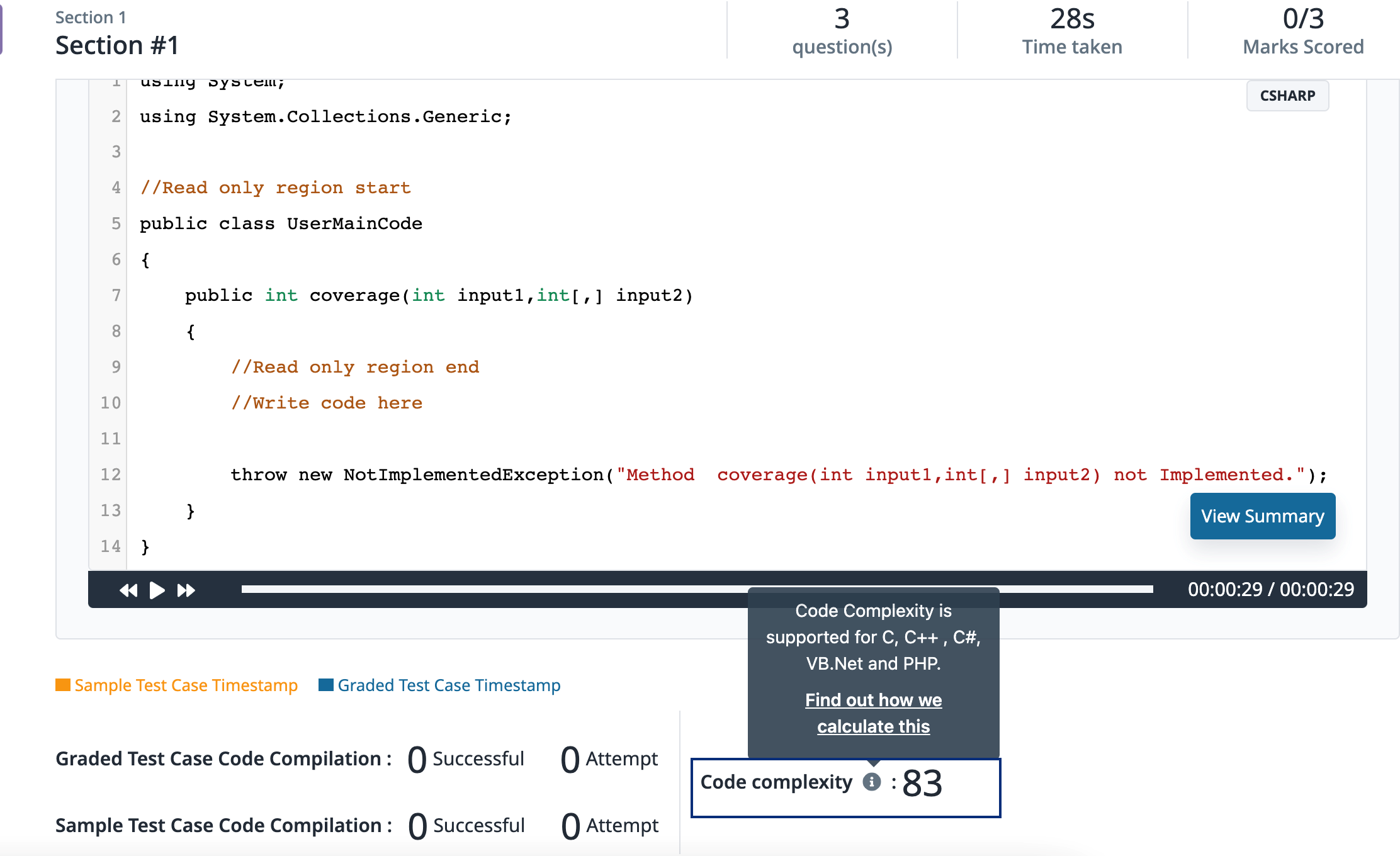# Code Complexity calculation on our Backend Programming Simulator

At Mercer | Mettl, the Code Complexity Score on CodeLysis is calculated as the inverse of a test-taker's Cyclomatic Complexity score. This score enables hiring managers to make better and more informed decisions that are not just based on the accuracy of the code written by the test-taker.

A test-taker’s report (who has attempted a code snippet question), contains the following details:
1. Total Marks scored by the test-taker
2. Coding Language Selected
3. Details of passed and failed test cases
4. Test-taker Code Playback
5. Code Complexity Score
What is Code Complexity and How is Code Complexity calculated

The Code Complexity score is the inverse of a test-taker's Cyclomatic Complexity score.

Cyclomatic Complexity is a software metric used to indicate the complexity of a program. It is a count of the number of decisions in the programmer’s code. Therefore, the higher the value, the more complex the code.Code Complexity is calculated as = 100 - Cyclomatic Complexity score

Hence, the less complex the test-taker’s code is, the higher will be their Code Complexity score and vice versa.

We at Mercer | Mettl currently support the calculation of Code
Complexity Score on our backend programming simulator i.e., CodeLysis for all coding languages except for the following:
1. C++
2. C#
3. VB.Net
4. PHP
For all other languages, the score would be currently reflected as 0.